# Quaternion.lookAt()

• anyone have thoughts on this? ive tried multiple ways with different variables and always seem to get a rotation of 45-53 degrees. new to unit vectors so maybe im just doing something wrong!

James1bow-owner

Rising World server's

(java) Wild Times Gaming

24.165.197.229:3250

(Unity) Wild Times Gaming

24.165.197.229:7254

https://discord.gg/CfNpxMkWxG

• What exactly do you want to do?
That the dragon always looks at you?
I'll think about it tomorrow, but for now it's just AFK:Heilige:

• What exactly do you want to do?
That the dragon always looks at you?

trying to get the dragon to look at a raycast position. so when command the dragons to move they look at the target while walking.

James1bow-owner

Rising World server's

(java) Wild Times Gaming

24.165.197.229:3250

(Unity) Wild Times Gaming

24.165.197.229:7254

https://discord.gg/CfNpxMkWxG

• I think I will repost here a simple solution that should help, it calculates quaternion from target position and original position:

Java
``````private Quaternion rotateTo(Vector3f objectPos, Vector3f lookPos) {
Vector3f dir = lookPos.subtract(objectPos).normalizeLocal();
float yaw = (float) Math.toDegrees(Math.atan2(dir.x, dir.z));
float pitch = (float) Math.toDegrees(Vector3f.UP.dot(dir));
return new Quaternion().fromAngles(pitch, yaw, 0);
}``````

Sometimes yaw can be inverted (depends on left/right axis rule used by game engine), in that case you can just swap x and z args. And if yaw has constant offset (for example 90 degrees) you can just add that value. Input of fromAngles method is in degrees. The last value in fromAngles is roll - rotation around direction axis

• The Quaternion.lookAt() method requires a direction (as unit vector), not a target position (that wouldn't work anyway, because a quaternion just describes a rotation, so it has no "start position"). If you have two vectors (e.g. your player position a and a target position b), and you want to get a vector from a to b, you can calculate b - a. In order to turn the resulting vector into a unit vector (i.e. a vector with a length of 1), you have to normalize it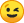So the code could look like this:

• morning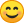I once found this post: https://stackoverflow.com/ques…uaternion-rotate-properly

First I got the Vector3f direction from the dragon to the point by Utils.VectorUtils.getDirection

Vector3f directionRot=Utils.VectorUtils.getDirection(dragon.getLocalPosition(),position.getPosition());

Then I created a multiplier and determined which axes would be used, only Y

Quaternion qFac = new Quaternion( 0, (float) Math.sin(PI/4), 0, (float) Math.cos(PI/4));

Next I get the quaternion direction of the dragon

Quaternion rRot = dragon.getLocalRotation().lookAt(directionRot);

Then the multiplier is multiplied by the quaternion

Quaternion fRot = qFac.multLocal(rRot);

Now the new viewing direction can be added to the dragon by creating a new quaternion, cropping the axes here again to be on the safe side, and normalizing the whole thing

dragon.setLocalRotation( new Quaternion(0, fRot.y, 0, fRot.w).normalizeLocal());

This is just how I calculate the direction for myself.
But I think if you adjust lines 12-18 and 21 it should work

• There is basically no need for this additional mathThe lookAt method already does all required calculations under the hood, the only "math" part on your end is to calculate the direction, which is targetVector - startVector, then normalize it. But I forgot that we already have a Utils method for that, as pointed out by noci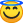Of course you can use that one instead of calculating the direction manually (just bear in mind that the first parameter is the "start position", and the 2nd parameter is the "target position")

This is a simplified version of my example above (using our utils method, but basically the utils method just performs the math posted above):

Java
``````//Get direction vector
Vector3f direction = Utils.VectorUtils.getDirection(dragonposition, result.getCollisionPoint);

//Get your new rotation looking at the direction
Quaternion rotation = new Quaternion().lookAt(direction);

//Let the dragon look at this position
dragon.setLocalRotation(rotation);``````
• Why this method is actually called lookAt (since there is a method with same name in Transform and it accepts target look point)? In quaternion there is a method called lookRotation, it is probably a better name for it since it clarifies that it doesn't look at position, but instead rotates to some specific direction

• Why this method is actually called lookAt (since there is a method with same name in Transform and it accepts target look point)? In quaternion there is a method called lookRotation, it is probably a better name for it since it clarifies that it doesn't look at position, but instead rotates to some specific direction

Basically we kept the method names from the Java version (to keep breaking changes as low as possible), and the old API was mostly using the naming convention of the engine (JMonkeyEngine), which also called it "lookAt"But actually I wouldn't recommend to rely too much on Unitys API when it comes to Vectors or Quaternions, because Unity works quite different in this regard anyway. Java provides no way to overload operators (so Unity code like "Vector3 c = a - b;" wouldn't work in Java), and in addition to that, Java doesn't have structs like C# - so creating new vectors or quaternions always result in a new object on the heap, which may have performance implications - that's why there are various "local" methods in the vector and quaternion classes, e.g. "add" (which always creates a new vector) and "addLocal" (which updates the original vector) etc.

The only API part which is directly related to Unity is Style (for UI elements). But apart from that, I wouldn't rely on Unitys API, because it is too different from the Plugin API.

• red51  noci  paulevs thank you all for the help! i was looking for some type of example and only found info on unity's Transform.lookAt() method, witch i assumed was correct. thank you all for the info as it makes alot more sense now!

James1bow-owner

Rising World server's

(java) Wild Times Gaming

24.165.197.229:3250

(Unity) Wild Times Gaming

24.165.197.229:7254

https://discord.gg/CfNpxMkWxG

• Java doesn't have structs like C# - so creating new vectors or quaternions always result in a new object on the heap

That's why Project Valhalla existsWell, if most names are related to original Java API that's fine, this should help old users to switch to newer API

• Ich stehe gerade auf dem Schlauch, vieleicht kann mir hier jemand helfen.

I'm at a loss right now, maybe someone here can help me.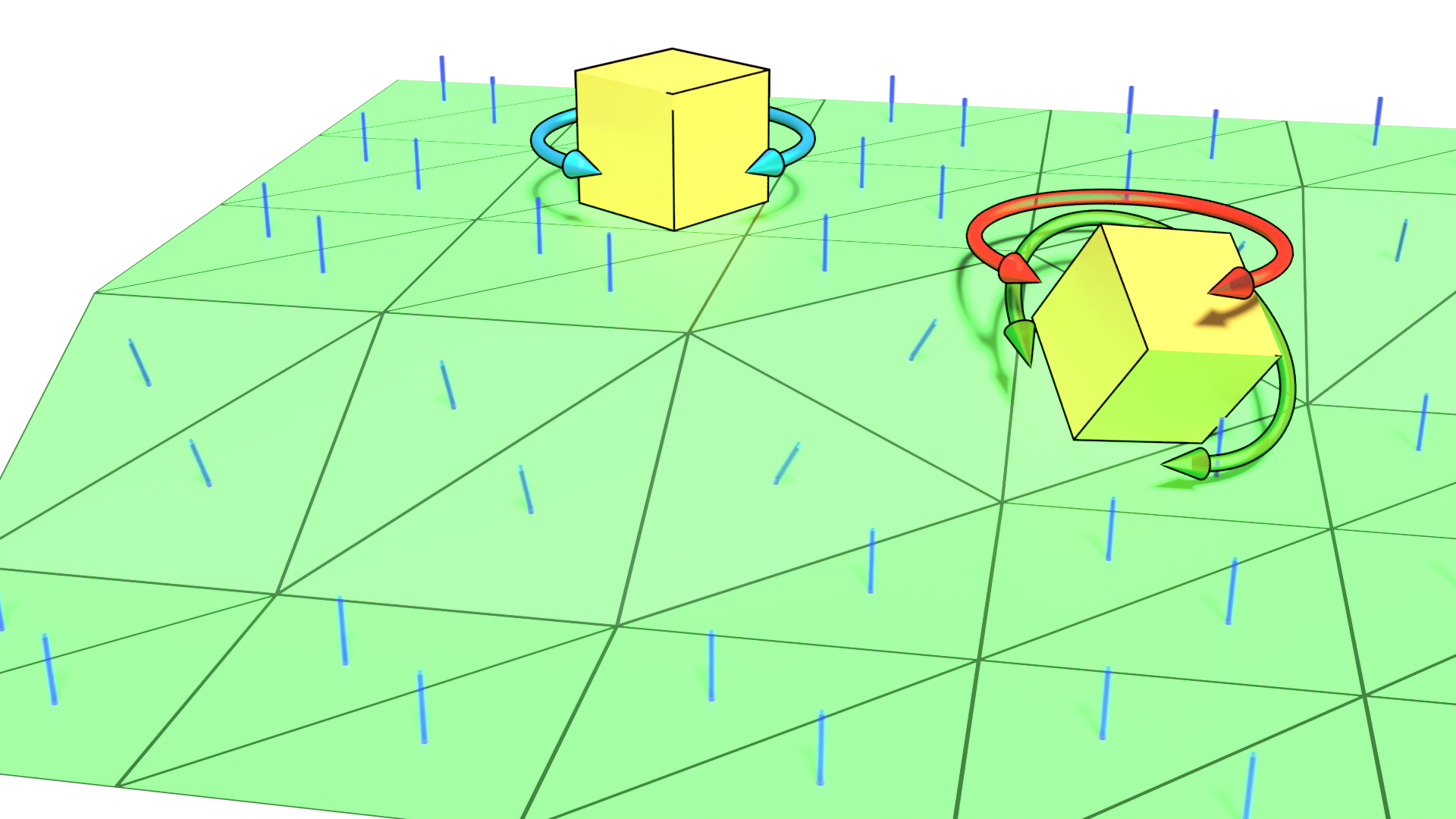Für die Situation der Blauen Rotation haben wir ja schon den weg gefunden.

We have already found the way to deal with the blue rotation situation.

Code
``````//Get direction vector
Vector3f direction = Utils.VectorUtils.getDirection(dragonposition, result.getCollisionPoint);

//Get your new rotation looking at the direction
Quaternion rotation = new Quaternion().lookAt(direction);

//Let the dragon look at this position
dragon.setLocalRotation(new Quaternion(0, rotation.y, 0, rotation.w));``````

Ich versuche jetzt die Grüne Rotation hin zu bekommen, sie soll sich an der Normale vom Terang ausrichten.
Allerdings finde ich da gerade kein Ansatz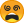Ich habe die Normale(Blaue Zylinder) als Vector3f und die Rote Rotarion als Quaternion.
Aktuell Dreht sich der Schräge Würfel um seine Ackse in der Roten Rotation, so das es eine von 360 blichrichtung gibt die Passt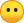I'm now trying to get the green rotation to align with the normal of the Terang.
However, I can't find an approach at the momentI have the normal (blue cylinder) as Vector3f and the red rotarion as quaternion.
Currently the slanted cube rotates around its axis in the red rotation, so that there is one of 360 light directions that fitsCode
``````Quaternion qFac = new Quaternion(
rayCast.getCollisionNormal().x*(float) Math.sin(PI/4f),
rayCast.getCollisionNormal().y*(float) Math.sin(PI/2f),
rayCast.getCollisionNormal().z*(float) Math.sin(PI/4f),
(float) Math.cos(PI/4f)
);
Prefab.setLocalRotation( qFac.multLocal( new Quaternion(0, rotation.y, 0, rotation.w) ));``````

## Participate now!

Don’t have an account yet? Create a new account now and be part of our community!